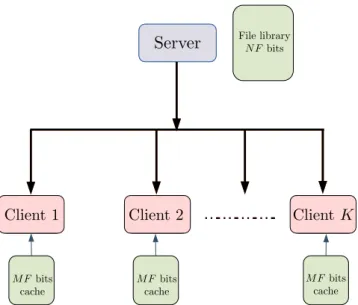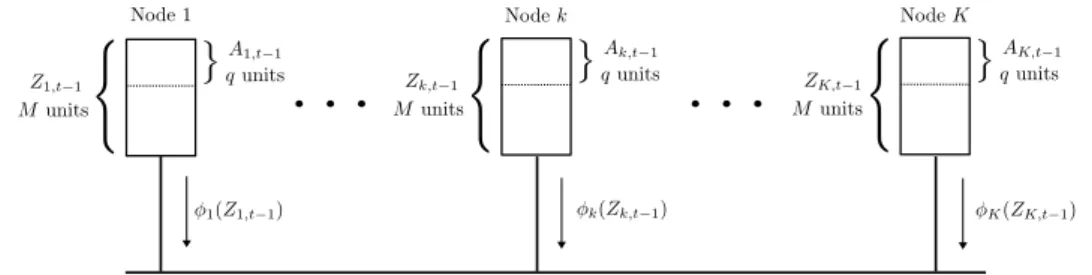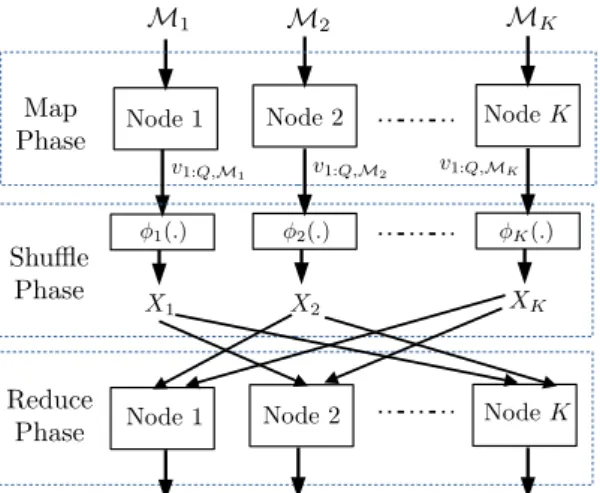# An Umbrella Converse for Data Exchange

N/A
N/A
Protected

Share "An Umbrella Converse for Data Exchange"

Copied!
32
0
0

Full text

We derived this lower bound independently in the conference version of this paper  and only recently learned of the bound in . In the coded caching system presented in , there is one server connected via a noiseless broadcast channel to Kclients indexed as [K]. In this section, we give another lower bound proof for coded caching derived in .

K}, where we give the index 0 to the server node and include it in the data exchange system. In the previous part of this section we showed the converse for the worst communication load L∗c for encrypted caching in the regime of K ≤ N. The caching and delivery schedule, as well as the optimal communication load L∗c, are defined as in the case of encrypted caching with homogeneous cache sizes.

This refinement basically follows similar subsequent steps as in the proof of Theorem 2 following (9), and finally leads to (22).Figure 1. A single server is connected to K clients via a broadcast channel. Each user has a cache capable of storing MF of the NF bits in the file-library available at the server.

## Decentralized Coded Data Shuffling

The above bound proved to be optimal for some special cases of the parameters, and order-optimal otherwise. The contents of the local cache for each node cat timet−1 is Zk,t−1, of which a subsetAk,t−1 is the active data currently being processed by the node. The worker nodes must communicate via a broadcast link to shuffle the active data between each other and create a new partition A1,t,.

We now recover the bound (26) by a simple proof using our generic lower bound in Theorem1. We assume that the cache placement and delivery scheme of the data shifting scheme is designed such that the communication load of the data shifting scheme is exactly equal to L∗ds. Application of Theorem1:Fork∈ [K]andQ⊂ [K], letAQk,denote the subset of bits of Ak,t that are available exactly at the nodes inQ and nowhere else.

Symmetrization by means of averaging over an appropriately chosen set of fluctuations: Let the set of circular permutations of K), apart from the identity permutation, be denoted by Γ. Refinement of the bound using set constraints and convexity: Now we have the following observations if AQk0,t−1:k0 ∈[K],{Q0 ⊂[K]:k0∈Q}forms a partition of all the NBbits. We considered the decentralized version of the coded data shuffling problem in this subsection.

A centralized version of the data mixing problem was introduced in  and its information-theoretic bounds were studied in detail in . Our data exchange binding when applied to the setting in  produces a looser inverse result than that in . The reasons for this are explored in Section 5 using the relation between our data exchange bound and the index coding bound known in the literature.Figure 2. The decentralized data shuffling problem. The contents of the local cache of each node k at time t − 1 is Z k,t−1 , out of which a subset A k,t−1 is the active data currently processed by the node.

## Coded Distributed Computing

The minimum communication load L∗d caused by a distributed computing system of K nodes for a given computational load r, where each reduction function is computed on exactly one node and each node computes WK reduction functions, is bounded by . A symmetrization step involving averaging over demand configurations is not applicable in the present setting because the definition of L∗dcin also involves minimization in the assignment of the reduction function. Recall that each reduction function is computed on exactly one node in our current setup.

To do this, we first denote by ~aQ the number of files whose intermediate outputs are required by some node and are exclusively available in the nodes in Q. Since the number of reduction functions assigned to nodekis WK (since each reduction function is computed at exactly one node) and each intermediate output is Tbits, is the number of intermediate output bits required by any node and available exclusively in the nodes of QareWTK a˜Q. For any Q⊂[K], the setneaQP in Theorem1 is thus given as follows. As in the previous case, we will denote the communication load for a given map feature assignment by LM(s) and the optimal communication load by computation loadsbyL∗dc(s).

The communication overhead corresponding to the assignment of the mapping function M when each reduction function needs to be computed on s nodes is lower bounded than . As before, we will denote by ˜aQ the number of files whose outputs of the mapping function are available exclusively at nodes Q. We will further denote the number of intermediate output bits required exclusively by nodes inP and available exclusively at nodes QbybQP.

Then, applying Theorem1, the lower bound on the communication load in terms of {bQP} is given by. For a given subsetPof size, the number of subsets of large size contained within P∪Q and containPare(s−lj. The number of intermediate output bits available exclusively from the nodes inPand inQ,bQP is given by bQP =a˜ QWT( . j s−l).

## Relation to Index Coding Lower Bound

The above lemma, together with some convexity arguments arising from the constraints imposed by the computational burden, can be used to prove a lower bound on L∗dc(s). We then show that the lower bound in (42) is actually equal to the generalized independence number α of this index coding problem. While α is equal to the maximum value of the bound in Proposition 3 for all permutations on [K], our bound in Theorem 4 is equal to the average value of the lower bound given in Proposition 3 for all permutations on [K].

If we average the right-hand side of (41) with respect to allγ, we obtain 1. Since the bound in Theorem 4 is obtained by averaging allγ, rather than maximizing over allγ, we conclude that in general is weaker than the α-bound of index coding. The two boundaries are equal if and only if the boundary in Proposition3 has the same value for each permutationγ.

Although generally weaker, we note that the bound of Theorem4 is easier to use than the α-bound. We now consider the class of unicast problems, i.e. problems where each bit is required by exactly one of the nodes. For unicast problems, the bound in Theorem4 is equal to L∗if and only if every S⊂[K] with|S| ≥2satisfies the following, cS\k{k}=cS\k{k0}0 for every k,k0 ∈S.

When the lower bound of Theorem4 is tight, the clique-coverage based index coding scheme (see in [26,27]) gives the optimal communication cost. For example, consider the simple canonical data mixing setting where the system has exactly K files, all equal Fbits, and each node stores exactly one of these files, i.e. the entire contents of thekth nodeCkis thekth file. Therefore, our lower bound is strictly less than L∗ for this data shuffling problem and is therefore not tight.

## Relationship to Other Index Coding Settings

Note that such integers∗J :J ∈ J will exist since every index coding problem has at least one solution, namely the trivial solution consisting of uncoded transmissions of xj :j∈[n]. Note that ∑J∈J s∗J is exactly the minimum number of bits that must be communicated by the servers to satisfy receiver requirements. Finally, we apply our data exchange bound in Statement1 to the distributed index encoding setting.

Any lower bound on the communication cost for this transformed setting with the virtual server will still apply to the original distributed setting with messages of length tj:j∈[n]. We now consider the embedded index coding problem, introduced in , motivated by device-to-device communications. The embedded index encoding setting consists of an array of data blocks (each a binary vector of length) distributed across an array of nodes.

Each node transmits a code word obtained by encrypting its data blocks, and each requested data block at each node is decoded from code words received from other nodes and side information at the node itself. The embedded index code consists of a collection of such encoding functions and decryption functions at the nodes, such that all required blocks are decrypted at the corresponding nodes. The work  generalizes the notion of minorrank of single-server index codes to determine the optimal length of linear embedded index codes.

Since the embedded index coding problem clearly has a direct mapping to the data exchange problem addressed in the present work, we can apply our data exchange bound directly to obtain a new lower bound on the communication cost of embedded index coding. The expression of this binding would be in the same form (up to only the change in notation) as the sentence itself. As our bottom is valid in an information theoretic sense, it would not only apply to the linear codes considered in , but also to nonlinear embedded index codes.

## Conclusions

Applying Theorem 1 and symmetrizing: As in the proof of Theorem 2, we use the index 0 to represent the server. Consider a demand vector d= (d1, ...,dK), such that the demands of the customers in A are different, i.e. di 6=dj. LetΩ consist of the N−1 cyclic permutations of(1, . . ,N), which are N-cycles, together with the identity permutation.

Without loss of generality, we assume that all caches are fully populated with file library bits (since we are constraining the optimal load), so we have ∑Q⊂[K]|Q|aQ=tNF. The choice of the set of demand vectors used for symmetry is the same as in Appendix B. Applying our main result, Theorem 1 to the subproblem caused by the demands of a subset of nodes A, we obtain.

LetI1=H, and note that since I1 is itself a subset of H, it must contain an information bit, for example wQP,m, requested by a node, for exampleπ1, and that none of the bits inI1\ {wQP,m} are available if side information onπ1. If Ik⊂ H it contains an information bit such that this bit is requested by a node, for example πk ∈[K]\ {π1,. We also note that for any choice of k0, none of the bits of ik0 are available as side information.

Ifk > k0, asIk ⊂ Ik0, we conclude that none of the bits in Ik are available as side information in πk0. Thus, we conclude that each bit in Ik\Ik+1 is required by πand is neither required nor available as side information in any of π1,. Therefore,|Ik\Ik+1|is bounded above by the number of bits required exclusively by πand possibly a subset of i{πk+1,.

For Theorem 4 to be tight, it is necessary that the limit of this theorem is equal to α, that is, the value of f is the same for all permutationsγ. If k0∈Q, then according to the induction hypothesis the term −ckQ∪k\k0 0 present in the third summation will cancel cQk.

FigureReferences

Related documents

2 hrs 2.5 Theory of organizational structures - nature and consequence of structure 2 hrs 3 Module B: Impact of structure, organization change and intervention strategy 3.1

The Central Valley Water Board, for example, addresses nitrate in groundwater through four programs:  irrigated lands, minimizing discharge from irrigated agricultural lands  CV

Source: Internet Figure 3: Oculus Quest 5 USES OF VIRTUAL REALITY FOR ARCHITECTS VISUALISATION, DESIGN, CLIENT COMMUNICATION, ENVIRONMENTAL DATA, EDUCATION 5.1 Visualization

Sanctioned date Amount ReceivedIn Rupees Amount received in words Dr.Ch.Shilpa Chakra IIIC TEQIP-II synthesis and characterization of nanomaterials for energy storage

Lyngdoh M 9856024199 Preservation and Processing of fruits and vegetables KVK Umdihar Meghalaya Ri-Bhoi Umsning Umdihar 165 Remius M 9856194720 Pig and poultry farming KVK Mawbri

IIT-H develops oil-based drug delivery system to fight fungal infections The new medication can even counter fungi that have developed resistance to conventional antifungal drugs

When a laser interacts with a plasma, the emitted photon energy is de- termined by the laser’s intensity, as well as target parameters such as thickness, atomic number, and the cross

ALL INDIA WEEKLY WEATHER REPORT 16 SEPTEMBER – 22 SEPTEMBER, 2021 Go to:-Table-1ATable-1B Table-1CTable-2 Table-3 Fig-1Fig-2Annexure-1 SIGNIFICANT WEATHER FEATURES ♦ A Well Marked

The observations show a multitude of blue, kilometer-scale, discharges at the cloud top layer at ~18 km altitude and a pulsating blue discharge propagating into the stratosphere

It can be observed that even under this scenario, the resulting network loss obtained using the proposed approach is close to the optimal value and the voltages at the PCC are close to